# Free Convection in a Vertical Slit Micro-channel with Super-hydrophobic Slip and Temperature Jump Conditions

Free Convection in a Vertical Slit Micro-channel with Super-hydrophobic Slip and Temperature Jump Conditions

Mani Ramanuja Gunduboina Gopi KrishnaHari Kamala Sree Vatukuru Naga Radhika

Department of Mathematics, Marri Laxman Reddy Institute of Technology &Management, Dundigal, Hyderabad 500043, India

Department of Mathematics, GITAM Institute of Technology and Management, Bangalore, Karnataka 561203, India

Department of H & S, Marri Laxman Reddy Institute of Technology, Dundigal, Hyderabad 500043, India

Corresponding Author Email:
gopikrishnagmsc@gmail.com
Page:
738-744
|
DOI:
https://doi.org/10.18280/ijht.380318
21 February 2020
|
Accepted:
28 August 2020
|
Published:
15 October 2020
| Citation

OPEN ACCESS

Abstract:

The main objective of the convective incompressible fluid flow placed in a magnetic field, with vertical motion through an isothermally heated porous channel. The Super-hydrophobic the slip was applied on one wall along with temperature jump, while there is no slip on another wall. The analytical results are discussed qualitatively for the parameters which were on the mathematical formulation on the basis of the model equations designed for the linear momentum and energy balance. Decreasing the value of the slip parameter is noticed due to decreasing in temperature. The effects of heat source and porous medium on the micro channel flow is analysized in detail. Coparesion of current results is made with the earlier work. The effect of Dray parameter is to increase the fluid velocity in the channel.

Keywords:

MHD, heat transfer, superhydrophobic slip, porous medium

1. Introduction

Based on the analytical study, a note is presented depicting an open-ended vertical comparable plate microchannel containing natural convection of a liquid in it which was fully-developed, where the thermal boundary and the hydrodynamic conditions differ on the dual walls of the channel. Superhydrophobic temperature and surface velocity slip bound are exhibited on one wall, while the other wall is normal. Comparison has been on superhydrophobic surface and non-slip surface due to heating where the temperature is constant.

The advancement of compact structure in technology in contour through the needs of people for smaller equipment and lighter appliances turns the inattention of scientists, engineers, and technologists towards researches, experimentations, and practical studies in mini-technology, then micro-technology, and later nano-technology. This is what caused researchers headed for work of momentum and heat transport behavior in macro-channels to mini-channels, micro-channels, and nano-channels.

Jah and Ajibade  studied the impact of viscous dissipation on natural convection folic in between vertical parallel plates and with periodic boundary conditions. Heat generation along with absorption effects on natural convection flow between two vertical parallel plates which are infinite are subjected to periodic heating in the presence of suction and injection were studied by Jha and Ajibade , as it was shown, the impact of heat generation is reciprocal to absorption on flow. Le et al.  are discussed and Modified the conditions for temperature jump and viscous heat generation. Ng and Wang  discussed about buoyancy-based flow in a vertical annular microchannel where the inner or either outer wall exhibits superhydrophobic velocity slip and also temperature jump, while the inner wall is maintained keeping a constant temperature. Kim  focused on operational design methodologies to arrive at the relevant dimensions for an optimal heat sink. Free convection flow of conducting fluid through a vertical parallel plate small scale divert within the limits of transverse attractive field is considered by Jha et al.  Wang and Chen  postulated a mathematical module for magneto gas dynamic convection within an isothermal planar microchannel under the influence of the applied electrical and magnetic field. Buonomo et al.  postulated an analytical solution for convection in the microchannel filled with a porous medium in the non-equilibrium condition. Wang  developed an analytical solution for thermo creep convection along the horizontal microchannel. Raghuraman et al.  conducted investigations of heat transfer and also the fluid flow properties in a rectangular microchannel heat sink. Narrien et al.  analyzed the transverse magnetic properties field on properties of heat transfer and fluid flow in a rectangular channel microchannel heat be submerged (MCHS). Tullius et al.  cooling conditions in micro-channels are discussed. Ma et al.  illustrated Generation analysis on fluid flow entropy for Al2O3-H2O nanofluid in microchannel plate heat sink. Torabi et al.  developed external boundary conditions in microporous channel, the generation rate of entropy principles varies with the parameter, temperature jump. Adesanya  illustrated the effect of various parameters of flows w.r.t the profiles of velocity temperature and. He studied the impact of the velocity slip along with temperature raise on the heat transfer and the skin friction. Wang and Ng  investigated on the temperature jump and concluded that it has a negative impact the flow rate on the superhydrophobic side of the channel at regular temperature, whereas it has a positive effect. when the heating is on the no-slip side of the channel. Çetin  developed the thermal systems with modified design inclusive of convective heat transfer in a microchannel operating at small Reynolds numbers. Anyanwu et al.  illustrated the importance of the Nusselt number at the two walls of the channel, which shows an unbounded swing which varies with an increase in parameters of fluid-wall interface and rarefaction. Li and Hrnjak  microchannel heat exchanger model was developed by taking into consideration the transport properties and thermodynamics of the refrigerant and oil combination very recently, Chamkha et al.  published their analytical, experimental and, numerical studies based on broad extensive assessment of nanofluid purposes in microchannels. Weng  modeled the developing convection in an asymmetrically heated planar microchannel based on the second-order Beskok–Karniadakis slip/jump boundary conditions by using a marching implicit procedure. Yu and Ameel  studied slip- ̄flow heat transfer in a micro-scale gap formed from isothermal surfaces. Contrary to all previous studies, axial conduction effect was included. Their results were similar to those of Larrodé et al.  however, the inclusion of axial conduction was found to increase heat transfer and the thermal entrance length. Öztop et al.  have performed a computational study of the thermal and dynamical behavior of fluid in an enclosure with two isothermal semi-circular heaters. They proved that the distance between the semi-circular heaters is the most important parameter affecting the heat and fluid flow fields. In addition, the Hartmann number was found to have an adverse effect on heat transfer. Rudraiah et al.  investigated numerically the effect of magnet-icefield on natural convection in a rectangular enclosure. They found that the magnetic field decreases the rate of heat transfer.

From the perspective of the above examinations, free convection in MHD flow through a porous medium, in a vertical slit microchannel with super-hydrophobic slip and temperature soar conditions with a heat source. The velocity and temperature are obtained. The impact of specific parameters on the flow quantities is explained through graphs.

2. Physical Model and Governing Equation

## 1.pngFigure 1. Physical configuration of flow diagram

For mathematical modelling, with the assumption that the flow is steady, incompressible, laminar, and totally developed. The two plates are separated by a distance h apart. The inducement of free-convection flow is due to a constant pressure gradient which is in an upward direction. The magnetic field strength B0 which is constant and the uniform was applied across the surface of the plates as shown in Figure 1. The x- axis represents the flow direction which is parallel to the gravity induced acceleration-g, whereas the y-axis which represents the flow rate is normal to it. The wall temperatures, T1 at the hotter plate (y=L) and T2 at cooler plate (y=0) are maintained.

The basic equations of the problem are taken from Jha and Gwandu .

Case I (The super-hydrophobic surface being heated)

The system followed the below governing equations:

$v \frac{d^{2} u}{d y^{l^{2}}}+g \beta_{0}\left(T-T_{0}\right)-\frac{\sigma B_{0}^{2} u}{\rho}-\frac{1}{K} u=0$  (1)

$K_{0} \frac{d^{2} T}{d y^{l^{2}}}+Q_{0}=0$  (2)

Their respective boundary conditions are:

$\left.\begin{array}{l}u\left(y^{l}\right)=\lambda^{l} \frac{d u}{d y^{l}} \\ T\left(y^{l}\right)=T_{h}+\gamma^{l} \frac{d T}{d y^{l}}\end{array}\right\}$ at $y^{l}=0$  (3)

$\left.\begin{array}{l}u\left(y^{l}\right)=0 \\ T\left(y^{\prime}\right)=T_{0}\end{array}\right\} \quad$ at $y^{l}=L$  (4)

Using the below dimensionless variables:

\begin{align} & \left( Y,\,\gamma ,\,\lambda \right)=\,\,\,({{y}^{\iota }},{{\gamma }^{\iota }},{{\lambda }^{\iota }})/L,\,\,\,\,\,\,\theta =(T-{{T}_{0}})/({{T}_{h}}-{{T}_{0}}) \\ & {{M}^{2}}=\sigma B_{0}^{2}{{L}^{2}}/pv,\,\,U=uv{{\left[ g{{\beta }_{0}}{{L}^{2}}({{T}_{h}}-{{T}_{0}}) \right]}^{-1}}, \\ & \beta =\frac{{{Q}_{0}}{{h}^{2}}}{{{K}_{0}}\left( {{T}_{h}}-{{T}_{0}} \right)} \\\end{align}   (5)

Eqns. (2) and (3) becomes:

$\frac{d^{2} U}{d y^{2}}+\theta-M^{2} U-\frac{1}{D a} U=0$  (6)

$\frac{d^{2} \theta}{d y^{2}}+\beta=0$  (7)

Their defined corresponding boundary situations are:

$\left.\begin{array}{l}\theta(y)=0 \\ U(Y)=0\end{array}\right\}$ at $Y=1$   (8)

$\left.\begin{array}{l}\theta(Y)=1+\gamma \frac{d \theta}{d y} \\ U(Y)=\lambda \frac{d u}{d y}\end{array}\right\}$ at $Y=0$  (9)

Case II (The no-slip surface being heated)

Governing equations are specified in conditions (1) & (2). Eq. (5) was utilized to transform them into Eqns. (6) and (7). We now solve Eqns. (6) and (7), sing the accompanying limit conditions.

$\left.\begin{array}{l}T\left(y^{l}\right)=T_{0}+\gamma^{l} \frac{d T}{d y^{l}} \\ u\left(y^{l}\right)=\lambda^{l} \frac{d u}{d y^{l}}\end{array}\right\}$ at $y^{l}=0$   (10)

$\left.\begin{array}{l}T\left(y^{\prime}\right)=T_{h} \\ u\left(y^{\prime}\right)=0\end{array}\right\}$ at $y^{l}=L$  (11)

$\left.\begin{array}{l}T(Y)=\gamma \frac{d \theta}{d Y} \\ u(Y)=\lambda \frac{d U}{d Y}\end{array}\right\}$ at $Y=0$  (12)

$\left.\begin{array}{l}T(Y)=1 \\ u(Y)=0\end{array}\right\} \quad$ at $Y=1$  (13)

3. Solution of the Problem

Case I:

On solving Eqns. (6)-(7) along with boundary conditions given by (8) and (9), velocity and temperature are obtained as

$\theta=-\frac{\beta y^{2}}{2}+c_{1} y+c_{2}$  (14)

\begin{align} & u(y)={{c}_{3}}{{e}^{\sqrt{s}y}}+{{c}_{4}}{{e}^{\sqrt[-]{s}y}}- \\ & \frac{p}{2({{M}^{2}}+\frac{1}{Da})}\left( {{y}^{2}}+\frac{2}{{{M}^{2}}+\frac{1}{Da}} \right)+ \\ & \frac{{{c}_{1}}y}{{{M}^{2}}+\frac{1}{Da}}+\frac{{{c}_{2}}}{{{M}^{2}}+\frac{1}{Da}} \\\end{align}   (15)

where,

$\frac{\left(\frac{\beta}{2}-1\right)}{\gamma+1}=c_{1}, c_{2}=1+\gamma \frac{\left(\frac{\beta}{2}-1\right)}{1+\gamma}$

$c_{4}=\frac{1}{\left[(\lambda \sqrt{s}-1) \mathrm{e}^{-\sqrt{s}}+(\lambda \sqrt{s}+1) e^{\sqrt{s}}\right]}$

$\left\{\begin{array}{l}\frac{\beta e^{\sqrt{s}}}{\left(M^{2}+\frac{1}{D a}\right)^{2}}-\frac{c_{2} e^{\sqrt{s}}}{M^{2}+\frac{1}{D a}}+\frac{\lambda c_{1} e^{\sqrt{s}}}{M^{2}+\frac{1}{D a}}+ \\ \left.\frac{\beta(\lambda \sqrt{s}-1)}{2\left(M^{2}+\frac{1}{D a}\right)}\left(1+\frac{2}{\left(M^{2}+\frac{1}{D a}\right.}\right)-\frac{c_{1}(\lambda \sqrt{s}-1)}{M^{2}+\frac{1}{D a}}-\frac{c_{2}(\lambda \sqrt{s}-1}{M^{2}+\frac{1}{D a}}\right)\end{array}\right\}$

{{c}_{3}}=\frac{1}{{{e}^{\sqrt{s}}}}\left\{ \begin{align} & -{{c}_{4}}{{e}^{-\sqrt{s}}}+\frac{\beta }{2({{M}^{2}}+\frac{1}{Da})}\left( 1+\frac{2}{({{M}^{2}}+\frac{1}{Da})} \right) \\ & -\frac{{{c}_{1}}}{{{M}^{2}}+\frac{1}{Da}}-\frac{{{c}_{2}}}{{{M}^{2}}+\frac{1}{Da}} \\\end{align} \right\}

Case II:

On solving Eqns. (6)-(7) along with boundary conditions given by (12) and (13), velocity and temperature are obtained as:

$\theta=-\frac{\beta y^{2}}{2}+c_{5} y+c_{6}$  (16)

\begin{align} & u(y)={{c}_{7}}{{e}^{\sqrt{s}y}}+{{c}_{8}}{{e}^{\sqrt[-]{s}y}}- \\ & \frac{p}{2({{M}^{2}}+\frac{1}{Da})}\left( {{y}^{2}}+\frac{2}{{{M}^{2}}+\frac{1}{Da}} \right)+ \\ & \frac{{{c}_{5}}y}{{{M}^{2}}+\frac{1}{Da}}+\frac{{{c}_{6}}}{{{M}^{2}}+\frac{1}{Da}} \\\end{align}   (17)

where,

$\frac{\left( \frac{\beta }{2}+1 \right)}{\gamma +1}={{c}_{5}}\,,\,\,\,{{c}_{6}}=\frac{\gamma }{1+\gamma }\,\left[ 1+\frac{\beta }{2} \right]$

$c_{7}=\frac{1}{\left[\left(1-\frac{\lambda}{\sqrt{s}}\right) \mathrm{e}^{-\sqrt{s}}-\left(1+\frac{\lambda}{\sqrt{s}}\right) e^{\sqrt{s}}\right]}$

$\left\{\begin{array}\frac{\beta e^{\sqrt{s}}}{\left(M^{2}+\frac{1}{D a}\right)^{2}}+\frac{c_{6} e^{\sqrt{s}}}{M^{2}+\frac{1}{D a}}-\frac{\lambda c_{5} e^{\sqrt{s}}}{M^{2}+\frac{1}{D a}}+\\\frac{\beta}{2\left(M^{2}+\frac{1}{D a}\right)}\left(1+\frac{2}{\left(M^{2}+\frac{1}{D a}\right.}\right)\left(1-\frac{\lambda}{\sqrt{s}}\right)-\\\frac{c_{5}}{M^{2}+\frac{1}{D a}}\left(1-\frac{\lambda}{\sqrt{s}}\right)-\frac{c_{6}}{M^{2}+\frac{1}{D a}}\left(1-\frac{\lambda}{\sqrt{s}}\right)\end{array}\right\}$

{{c}_{8}}=\frac{1}{{{e}^{\sqrt{s}}}}\left\{ \begin{align} & -{{c}_{8}}{{e}^{-\sqrt{s}}}+\frac{\beta }{2({{M}^{2}}+\frac{1}{Da})}\left( 1+\frac{2}{({{M}^{2}}+\frac{1}{Da})} \right) \\ & -\frac{{{c}_{5}}}{{{M}^{2}}+\frac{1}{Da}}-\frac{{{c}_{6}}}{{{M}^{2}}+\frac{1}{Da}} \\\end{align} \right\}

4. Results and Discussion

The solution to the problem is computed by applying analytically. Computation was done using velocity and also temperature profiles variations and different values of corresponding parameters are displayed. The effects of different physical parameters like Hartmann number M, heat sourceβ, Darcy number Da and slip conditions λ and γ. The graphical discussion of fluid velocity and temperature were done.

Case I:

From Figure 2, we can observe the rise in the Hartmann’s number, suppresses the velocity field. However, we can conclude that the raise in the value of M leads to the reduction in velocity and makes the distribution more symmetrical. It can be concluded that the effect of the magnetic field plays a dampening role in MGD transport because of the magnetic body force in the momentum equation and creates a flatter velocity profile. Figure 3 display the have an effect on of porosity parameter Da on the velocity profiles for slip conditions λ=1 and γ=1.It is the velocity that increases increasing Darcy parameter Increases. From Figure 4 velocity profile decreases with increasing slip parameter γ. The increases heat source β increases the fluid velocity, which are presented in Figure 5. The difference in temperature θ with y is determined from condition (14) from various of estimations of β and appeared in Figure 6. The increments in the warmth source delivered the warmth, which upgraded the temperature profiles. Figure 7 illustrates that temperature decreases with an increase temperature jump coefficient.

## 2.jpg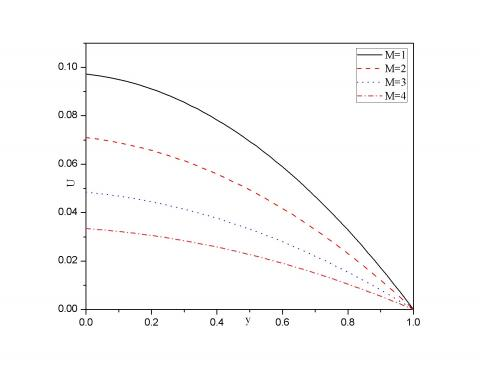Figure 2. Velocity profiles versus magnetic field variation M

## 3.jpgFigure 3. Velocity profiles versus Darcy number variation Da

## 4.jpg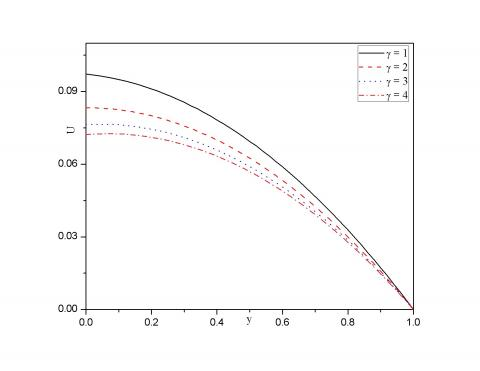Figure 4. Velocity profiles versus slip length variation γ

## 5.jpgFigure 5. Velocity profiles versus heat source coefficient variation β

## 6.jpgFigure 6. Temperatures profiles versus heat source coefficient variation β

Case II:

The difference of temperature θ with y is determined slip γ=1 from condition (16) from various estimations of β shown in Figure 8. The increases in the heat source produced the heat, which enhanced the temperature profiles. The variation of temperature θ with y is determined to slip γ=1 from condition (16) from various estimations of β shown in Figure 9. The effect of heat source produced heat, which enhanced the temperature profiles.

## 7.jpgFigure 7. Temperatures profiles versus Slip length variation γ

## 8.jpg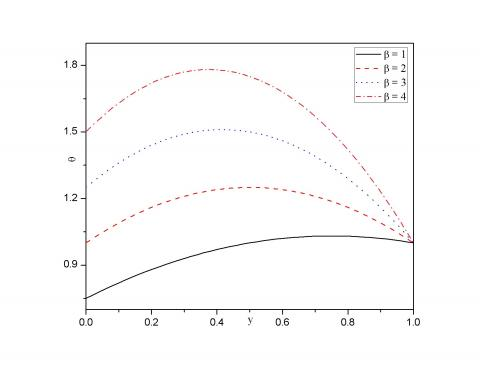Figure 8. Temperatures profiles versus heat source coefficient variation β

## 9.jpg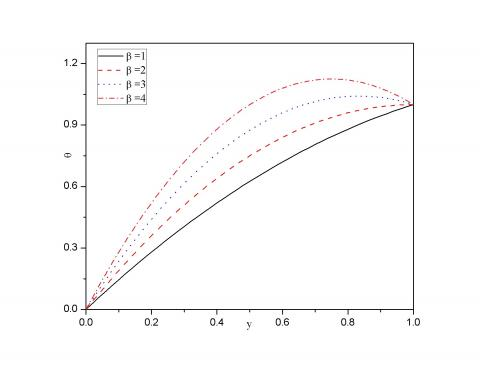Figure 9. Temperatures profiles versus heat source coefficient variation β

## 10.jpg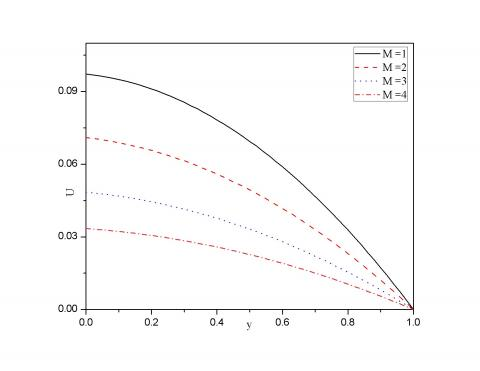Figure 10. Velocity profiles versus magnetic field variation M

## 11.jpg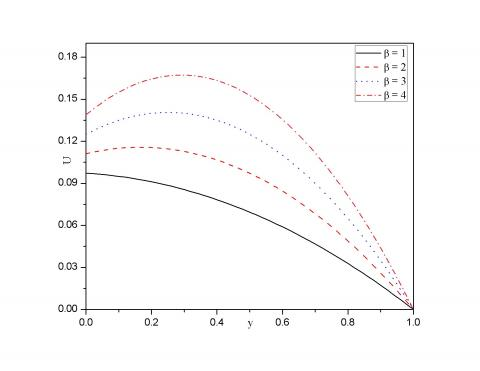Figure 11. Velocity profile versus heat source coefficient variation β

## 12.jpgFigure 12. Velocity profile versus Darcy number variation Da

It depicts from Figure 10 that the velocity decreases with rising Hartmann number M, because in the increases the magnetic field reduced the velocity. The effect of heat source on β is presented in Figure 11. It is obvious that the temperature source β enhanced the velocity profiles. Figure 12 showcase the impact of porosity parameter Da on velocity profiles for slip conditions λ=1 and γ=1. It is the velocity that will increases increasing Darcy parameter increases.

Table 1 descries a comparison of the current results with the already published work of Jha et al.  in the limiting sense. It is noticed that there is a good correlation between both the results.

Table 1. Comparison of volume flow rate in the limiting case when λ=γ=1

 M Present Work $\beta =0.5,Da=0.01$ Jha et al.  2016 1.5 1.8036 0.3403 2 1.8695 0.8750 2.5 1.9561 1.4825 3 2.0651 2.1944
5. Conclusion

This paper explored free convection in MHD flow through a permeable medium in a micro-channel (Vertical Slit) with super-hydrophobic slip and temperature bounce conditions with a constant heat source. The effect of various physical parameters on temperature and velocity are discussed through graphs. However, the conclusive remarks are laid down as;

Reduction in the velocity profiles is marked due to the attenuation of Hartmann number.

• The validation of the present result with earlier study shows a road map for further investigation.
• Remarkable enhancement is observed in both the velocity and temperature distribution for the increasing heat source.
• The temperature drops with increasing values of slip parameter γ. The same behavior is observed in velocity profile also.
• At constant slip conditions λ=1 and γ=1 velocity increase was observed with increase of porosity parameter Da.
Nomenclature
 u The dimensional upward velocity of the fluid flow yl Dimensional horizontal position in connecting the plates g Gravity induced acceleration T Temperature on the heated surface T0 Temperature of the surrounding Th Temperature higher than T0 B0 Magnetic field intensity Y Horizontal position U Upward velocity L Channel width M Magnetic field (parameter) Da Darcy number λ Slip length γ Slip length ν kinematic viscosity ρ Fluid density σ Fluid’s electrical conductivity β Heat source coefficient
References

 Jha, B.K., Ajibade, A.O. (2012). Effect of viscous dissipation on natural convection flow between vertical parallel plates with time-periodic boundary conditions. Communications in Nonlinear Science and Numerical Simulation, 17(4): 1576-1587. https://doi.org/10.1016/j.cnsns.2011.09.020

 Jha, B.K., Ajibade, A.O. (2009). Free convective flow of heat generating/absorbing fluid between vertical porous plates with periodic heat input. International Communications in Heat and Mass Transfer, 36(6): 624-631. https://doi.org/10.1016/j.icheatmasstransfer.2009.03.003

 Le, N.T., Tran, N.H., Tran, T.N. (2018). Modified Patterson temperature jump condition considering viscous heat generation. International Journal of Heat and Mass Transfer, 126: 1267-1274. https://doi.org/10.1016/j.ijheatmasstransfer.2018.06.046

 Ng, C.O., Wang, C.Y. (2014). Natural convection in a vertical microannulus with superhydrophobic slip and temperature jump. Journal of Thermophysics and Heat Transfer, 28(2): 287-294. https://doi.org/10.2514/1.T4200

 Kim, S.J. (2004). Methods for thermal optimization of microchannel heat sinks. Heat transfer engineering, 25(1): 37-49. https://doi.org/10.1080/01457630490248359

 Jha, B.K., Aina, B., Ajiya, A.T. (2015). MHD natural convection flow in a vertical parallel plate microchannel. Ain Shams Engineering Journal, 6(1): 289-295. https://doi.org/10.1016/j.asej.2014.09.012

 Weng, H.C., Chen, D.C. (2013). Magnetogasdynamic flow and heat transfer in a microchannel with isothermally heated walls. International Journal of Heat and Mass Transfer, 57(1): 16-21. https://doi.org/10.1016/j.ijheatmasstransfer.2012.10.003

 Buonomo, B., Manca, O., Lauriat, G. (2014). Forced convection in micro-channels filled with porous media in local thermal non-equilibrium conditions. International Journal of Thermal Sciences, 77: 206-222. https://doi.org/10.1016/j.ijthermalsci.2013.11.003

 Weng, H.C. (2016). Combined forced and thermocreep convection through a long horizontal microchannel. Micromachines, 7(2): 33. https://doi.org/10.3390/mi7020033

 Raghuraman, D.R.S., Raj, R.T.K., Nagarajan, P.K., Rao, B.V.A. (2017). Influence of aspect ratio on the thermal performance of rectangular shaped micro channel heat sink using CFD code. Alexandria Engineering Journal, 56(1): 43-54. https://doi.org/10.1016/j.aej.2016.08.033

 Narrein, K., Sivasankaran, S., Ganesan, P. (2016). Influence of transverse magnetic field on microchannel heat sink performance. Journal of Applied Fluid Mechanics, 9(6): 3159-3166.

 Tullius, J.F., Vajtai, R., Bayazitoglu, Y. (2011). A review of cooling in microchannels. Heat Transfer Engineering, 32(7-8): 527-541. https://doi.org/10.1080/01457632.2010.506390

 Ma, H., Duan, Z., Su, L., Ning, X., Bai, J., Lv, X. (2019). Fluid flow and entropy generation analysis of Al2O3–water nanofluid in microchannel plate fin heat sinks. Entropy, 21(8): 739. https://doi.org/10.3390/e21080739

 Torabi, M., Zhang, Z., Peterson, G.P. (2017). Interface entropy generation in micro porous channels with velocity slip and temperature jump. Applied Thermal Engineering, 111: 684-693. https://doi.org/10.1016/j.applthermaleng.2016.09.148

 Adesanya, S.O. (2015). Free convective flow of heat generating fluid through a porous vertical channel with velocity slip and temperature jump. Ain Shams Engineering Journal, 6(3): 1045-1052. https://doi.org/10.1016/j.asej.2014.12.008

 Wang, C.Y., Ng, C.O. (2014). Natural convection in a vertical slit microchannel with superhydrophobic slip and temperature jump. Journal of Heat Transfer, 136(3): 034502. https://doi.org/10.1115/1.4025822

 Çetin, B. (2013). Effect of thermal creep on heat transfer for a two-dimensional microchannel flow: An analytical approach. Journal of Heat Transfer, 135(10): 101007. https://doi.org/10.1115/1.4024504

 Anyanwu, E.O., Raymond, D., Okorie, C.E., James, F.E., Ogbaji, E.O. (2018). Slip boundary condition effects on the rate of heat transfer in a micro channel including viscous dissipation. International Journal of Scientific Research in Mathematical and Statistical Sciences, 5(4): 9-21. https://doi.org/10.26438/ijsrmss/v5i4.921

 Li, H., Hrnjak, P. (2015). An experimentally validated model for microchannel heat exchanger incorporating lubricant effect. International Journal of Refrigeration, 59: 259-268. https://doi.org/10.1016/j.ijrefrig.2015.07.020

 Chamkha, A.J., Molana, M., Rahnama, A., Ghadami, F. (2018). On the nanofluids applications in microchannels: A comprehensive review. Powder Technology, 332: 287-322. https://doi.org/10.1016/j.powtec.2018.03.044

 Weng, H.C. (2006). Developing natural convection with thermal creep in a vertical microchannel. Journal of Physics D: Applied Physics, 39(14): 3107. https://doi.org/10.1088/0022-3727/39/14/034

 Yu, S.P., Ameel, T.A. (2002). Slip flow convection in isoflux rectangular microchannels. Journal of Heat Transfer, 124(2): 346-355 https://doi.org/10.1115/1.1447932

 Larrodé, F.E., Housiadas, C., Drossinos, Y. (2000). Slip-flow heat transfer in circular tubes. International Journal of Heat and Mass Transfer, 43(15): 2669-2680. https://doi.org/10.1016/S0017-9310(99)00324-5

 Öztop, H.F., Rahman, M.M., Ahsan, A., Hasanuzzaman, M., Saidur, R., Al-Salem, K., Rahim, N.A. (2012). MHD natural convection in an enclosure from two semi-circular heaters on the bottom wall. International Journal of Heat and Mass Transfer, 55(7-8): 1844-1854. https://doi.org/10.1016/j.ijheatmasstransfer.2011.11.037

 Rudraiah, N., Barron, R.M., Venkatachalappa, M., Subbaraya, C.K. (1995). Effect of a magnetic field on free convection in a rectangular enclosure. International Journal of Engineering Science, 33(8): 1075-1084. https://doi.org/10.1016/0020-7225(94)00120-9

 Jha, B.K., Gwandu, B.J. (2018). MHD free convection flow in a vertical slit micro-channel with super-hydrophobic slip and temperature jump: Heating by constant wall temperature. Alexandria Engineering Journal, 57(4): 2541-2549. https://doi.org/10.1016/j.aej.2017.08.022